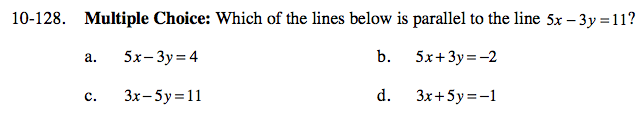### Home > CCA > Chapter 10 > Lesson 10.3.2 > Problem10-128

10-128.
1. Multiple Choice: Which of the lines below is parallel to the line 5x − 3y = 11? Homework Help ✎

1. 5x − 3y = 4

2. 5x + 3y = − 2

3. 3x − 5y = 11

4. 3x + 5y = − 1Write each equation in y = mx + b form, including the original equation.
Which one has the same slope as the original equation?